# Scalar quantities, phasor concept

## Introduction

The available quantities for post-processing can be scalar or vector quantities.

This section deals with the scalar quantities and recalls several definitions: rotating vector, complex image, phasor…

## Rotating vector, complex image

A sinusoidal time varying scalar quantity of pulsation ω can be geometrically represented by a vector rotating at the angular velocity ω.

This vector is the geometric representation of a complex number.

## Example: sinusoidal current and complex image

Let there be a sinusoidal time varying current i(t) of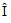amplitude, of ω pulsation (ω = 2 π f) and of β phase at the time t = 0.

The instantaneous value of this current, i : i = Î.sin(ω.t + β)

is equal to the imaginary part of the complex number i : i = Im(i)

Rotating vector with ω velocity

Sinusoidal quantity i(t) of period T=2 π/ωThe complex instantaneous value of a sinusoidal current is given by the following relation:

• under a cartesian form: i = Î.cos(ω.t + β) + j.Î.sin(ω.t+ β)
• under an exponential form: i = Î.e j(ω.t+ β)

where:

•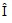is the modulus of the complex value i
• ωt + β is the argument (or the phase) of the complex value i
• β is the initial phase

## Complex notation of a rotating vector

The rotating vector assigned to the sinusoidal quantity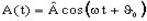is expressed under the form of a complex number A. This complex number can be written:

• under a carthesian form: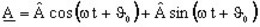• under an exponential form: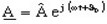## Phasor concept

The complex function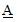associated to the sinusoidal quantity A(t) can be decomposed into two factors as follows: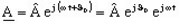This decomposition is presented in the table below.

The factor … which can be written … Corresponds to a …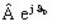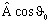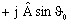rotating vector associated to the quantity A(t) at t = 0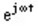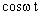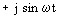vector rotation by an angle ωt
• The factor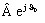contains information concerning the amplitude and the initial phase of the quantity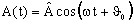• The factor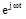contains the information concerning the time variation of the quantity

## Phasor concept

The sinusoidal function in the time domain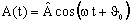can be represented in the complex domain by the phasor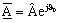.

## Phasor: definition

We call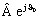the phasor assigned to the quantity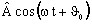, i.e the phasor associated to the quantityis the rotating vector associated with this quantity at a time t=0.